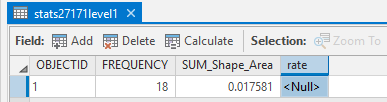# Calculate a field based on sum of a field from another shape file

529
5
03-26-2019 07:55 PMNew Contributor

Hi guys,

I need to calculate the field "rate" based on "SUM_Shape_Area" with the sum of "Shape_Area" in the second screenshot. So, the formula is "rate = SUM_Shape_Area/sum of Shape_Area". And I want to calculate the sum of Shape_Area first and then export it as a variable, and then use "arcpy.CalculateField_management" to calculate the "rate" field I need. I looked at different methods about how to calculate the sum of the field, but they did not work in my case. Could anyone help me with this? Any kind of help would be appreciated.Tags (2)
5 RepliesbyMVP Esteemed Contributor

Your big problem is that the units of area in that file are in square degrees which is pretty useless since it varies latitudinally. as for the division by area, that would be a fixed parameter, once convertedNew Contributor III

Hello Zhenyu Yao‌,

In your ArcGIS Pro, navigate to the Analysis tab, and click on python option.

A window will open, update the paths in the script below and paste it in the python window.

fc = r"path/to/the/table/containting/multiple/ShapeAreaValues"
summed_total = 0
with arcpy.da.SearchCursor(fc, "Shape_Area") as cursor:
for row in cursor:
summed_total = summed_total + row

fc_to_update = r"path/to/the/table/to/be/updated"
fields = ['SUM_Shape_Area', 'rate']
where_clause = "OBJECTID = 1"
with arcpy.da.UpdateCursor(fc, fields,where_clause) as cursor:
for row in cursor:
row = row / summed_total
cursor.updateRow(row)

Please let me know if you get any errors.byMVP Esteemed Contributor

You need to throw the conversion from square decimal degrees to projected planar units in otherwise the result will still be in square degrees to the sum of squared degreesNew Contributor III

How about changing the projection to PCS get the values in planar units and then run the script?byMVP Esteemed Contributor

doesn't produce a new file and enables adding a field of areas in a specified projection.  You could then add a new field and do the calculation

A cursor could then be used, but it often quicker to use numpy to get the column out as an array, do the sum and the ratio, then arcpy.da.ExtendTable to add a new field with the results back